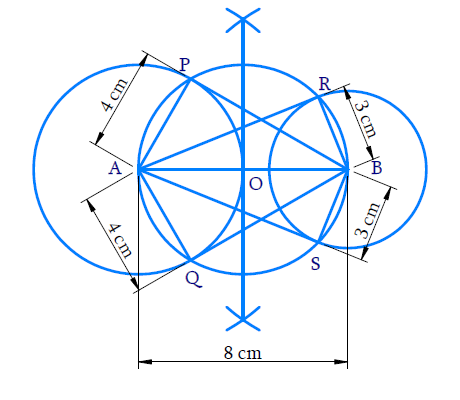# Ex.11.2 Q5 Constructions Solution - NCERT Maths Class 10

Go back to  'Ex.11.2'

## Question

Draw a line segment $$AB$$ of length $$8 \,\rm{cm}$$. Taking $$A$$ as centre, draw a circle of radius $$4 \,\rm{cm}$$ and taking $$B$$ as center, draw another circle of radius $$3 \,\rm{cm}$$. Construct tangent to each circle from the center of the other circle.

Video Solution
Constructions
Ex 11.2 | Question 5

## Text Solution

#### Steps:Steps of construction:

(i) Draw \begin{align}\rm{AB}=8\, \rm{cm}\end{align}.With $$A$$ and $$B$$ as centers $$4 \,\rm{cm}$$ and $$3 \,\rm{cm}$$ as radius respectively draw two circles.

(ii)  Draw the perpendicular bisector of $$AB$$, intersecting $$AB$$ at $$O$$.

(iii) With $$O$$ as center and $$OA$$ as radius draw a circle which intersects the two circles at $$P$$, $$Q$$, $$R$$ and $$S$$.

(iv) Join $$BP$$, $$BQ$$, $$AR$$ and $$AS$$.

(v) $$BP$$ and $$BQ$$ are the tangents from $$B$$ to the circle with center $$A$$$$AR$$ and $$AS$$ are the tangents from $$A$$ to the circle with center $$B$$.

Proof:

$$\angle {APB}=\angle {AQB}=90^{\circ}$$ (Angle in a semi-circle)

$$\therefore \rm{AP} \perp \rm{PB}$$ and $${AQ} \perp {QB}$$

Therefore, $$BP$$ and $$BQ$$ are the tangents to the circle with center $$A$$.

Similarly, $$AR$$ and $$AS$$ are the tangents to the circle with center $$B$$.

Learn from the best math teachers and top your exams

• Live one on one classroom and doubt clearing
• Practice worksheets in and after class for conceptual clarity
• Personalized curriculum to keep up with school